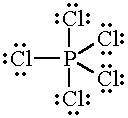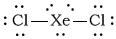# Do all of the following molecules contain at least one bond angle at 120 degrees: SeS3, SeS2, PCl5, TeCl4, ICI3, and XeCl2?

Feb 9, 2016

No, because two of the molecules have no bond angles at 120 °.

#### Explanation:

To answer this question, you must draw the Lewis structures of the compounds and determine their VSEPR shapes.

${\text{SeS}}_{3}$

The Lewis structure of ${\text{SeS}}_{3}$ is(from www.homeworklib.com)

This is an ${\text{AX}}_{3}$ molecule, and its geometry is trigonal planar.

All bond angles in a trigonal planar molecule are approximately 120 °.

${\text{SeS}}_{2}$

The Lewis structure of ${\text{SeS}}_{2}$ is

$: \stackrel{\text{. .")("S")=stackrel(". .")("Se")=stackrel(". .")("S}}{:}$

This is an $\text{AX"_2"E}$ molecule.

Its electron geometry is trigonal planar.

The bond angle in an $\text{AX"_2"E}$ molecule is approximately 120 °.

${\text{PCl}}_{5}$

The Lewis structure of ${\text{PCl}}_{5}$ isThis is an ${\text{AX}}_{5}$ molecule, and its geometry is trigonal pyramidal.

The equatorial bond angles in a trigonal bipyramidal molecule are all 120 °.

${\text{TeCl}}_{4}$

The Lewis structure of ${\text{TeCl}}_{4}$ isThis is an $\text{AX"_4"E}$ molecule.

Its electron geometry is trigonal pyramidal.

The equatorial bond angle between the equatorial $\text{Te-Cl}$ bonds is approximately 120 °.

${\text{ICl}}_{3}$

The Lewis structure of ${\text{ICl}}_{3}$ isThis is an ${\text{AX"_3"E}}_{2}$ molecule.

Its electron geometry is trigonal pyramidal, and its molecular geometry is T-shaped.

The $\text{I-Cl}$ bond angles in a T-shaped molecule are both approximately 90 °.

${\text{XeCl}}_{2}$

The Lewis structure of ${\text{XeCl}}_{2}$ isThis is an ${\text{AX"_2"E}}_{3}$ molecule.

Its electron geometry is trigonal pyramidal, with the lone pairs in the equatorial positions.

The molecule is linear, with a $\text{Cl-Xe-Cl}$ bond angle of 180 °.